Theory and Modern Applications

# On stability of discrete-time systems under nonlinear time-varying perturbations

## Abstract

We give some explicit stability bounds for discrete-time systems subjected to time-varying and nonlinear perturbations. The obtained results are extensions of some well-known results in (Hinrichsen and Son in Int. J. Robust Nonlinear Control 8:1169-1188, 1998; Shafai et al. in IEEE Trans. Autom. Control 42:265-270, 1997) to nonlinear time-varying perturbations. Two examples are given to illustrate the obtained results. Finally, we present an Aizerman-type conjecture for discrete-time systems and show that this conjecture is valid for positive systems.

MSC:39A30, 93D09.

## 1 Introduction and preliminaries

Discrete-time equations have numerous applications in science and engineering. They are used as models for a variety of phenomena in the life sciences, population biology, computing sciences, economics, etc.; see, e.g., [6, 10, 15].

Motivated by many applications in control engineering, problems of stability and robust stability of dynamical systems have attracted much attention from researchers for a long time, see, e.g., [2, 3, 5, 911, 1421] and references therein. In this paper, we investigate exponential stability of discrete-time systems subjected to nonlinear time-varying perturbations. Some explicit stability bounds for discrete-time systems subjected to nonlinear time-varying perturbations are given. Furthermore, we present an Aizerman-type conjecture for discrete-time systems and show that it is valid for positive systems. Two examples are given to illustrate the obtained results.

Let $\mathbb{R}$ be the set of all real numbers and let $\mathbb{N}$ be the set of all natural numbers. Set ${\mathbb{Z}}^{+}:=\mathbb{N}\cup \left\{0\right\}$. For given $N\in \mathbb{N}$, let us denote $\underline{N}:=\left\{1,2,\dots ,N\right\}$. Let n, l, q be positive integers. Inequalities between real matrices or vectors will be understood componentwise, i.e., for two real $l×q$-matrices $A=\left({a}_{ij}\right)$ and $B=\left({b}_{ij}\right)$, the inequality $A\ge B$ means ${a}_{ij}\ge {b}_{ij}$ for $i=1,\dots ,l$; $j=1,\dots ,q$. In particular, if ${a}_{ij}>{b}_{ij}$ for $i=1,\dots ,l$; $j=1,\dots ,q$, then we write $A\gg B$ instead of $A\ge B$. The set of all nonnegative $l×q$-matrices is denoted by ${\mathbb{R}}_{+}^{l×q}$. If $x=\left({x}_{1},{x}_{2},\dots ,{x}_{n}\right)\in {\mathbb{R}}^{n}$ and $P=\left({p}_{ij}\right)\in {\mathbb{R}}^{l×q}$ we define $|x|=\left(|{x}_{i}|\right)$ and $|P|=\left(|{p}_{ij}|\right)$. It is easy to see that $|CD|\le |C||D|$. For any matrix $A\in {\mathbb{R}}^{n×n}$ the spectral radius of A is denoted by $\rho \left(A\right)=max\left\{|\lambda |:\lambda \in \sigma \left(A\right)\right\}$, where $\sigma \left(A\right):=\left\{z\in \mathbb{C}:det\left(z{I}_{n}-A\right)=0\right\}$ is the set of all eigenvalues of A. A norm $\parallel \cdot \parallel$ on ${\mathbb{R}}^{n}$ is said to be monotonic if $|x|\le |y|$ implies $\parallel x\parallel \le \parallel y\parallel$ for all $x,y\in {\mathbb{R}}^{n}$. Every p-norm on ${\mathbb{R}}^{n}$ (${\parallel x\parallel }_{p}={\left({|{x}_{1}|}^{p}+{|{x}_{2}|}^{p}+\cdots +{|{x}_{n}|}^{p}\right)}^{\frac{1}{p}}$, $1\le p<\mathrm{\infty }$ and ${\parallel x\parallel }_{\mathrm{\infty }}={max}_{i=1,2,\dots ,n}|{x}_{i}|$), is monotonic. Throughout this paper, the norm $\parallel M\parallel$ of a matrix $M\in {\mathbb{R}}^{l×q}$ is always understood as the operator norm defined by $\parallel M\parallel ={max}_{\parallel y\parallel =1}\parallel My\parallel$ where ${\mathbb{R}}^{q}$ and ${\mathbb{R}}^{l}$ are provided with some monotonic vector norms. Then, the operator norm $\parallel \cdot \parallel$ has the following monotonicity property (see, e.g., )

$P\in {\mathbb{R}}^{l×q},Q\in {\mathbb{R}}_{+}^{l×q},\phantom{\rule{1em}{0ex}}|P|\le Q\phantom{\rule{1em}{0ex}}⇒\phantom{\rule{1em}{0ex}}\parallel P\parallel \le \parallel |P|\parallel \le \parallel Q\parallel .$
(1)

The next theorem summarizes some basic properties of nonnegative matrices which will be used in what follows.

Theorem 1.1 ([8, 12])

Let$A\in {\mathbb{R}}^{p×p}$be a nonnegative matrix. Then the following statements hold.

1. (i)

(Perron-Frobenius Theorem) $\rho \left(A\right)$ is an eigenvalue of A and there exists a nonnegative eigenvector $x\in {\mathbb{R}}^{p}$, $x\ne 0$ such that $Ax=\rho \left(A\right)x$.

2. (ii)

Given $\alpha \in {\mathbb{R}}_{+}$, there exists a nonzero vector $x\ge 0$ such that $Ax\ge \alpha x$ if and only if $\rho \left(A\right)\ge \alpha$.

3. (iii)

${\left(t{I}_{n}-A\right)}^{-1}$ exists and is nonnegative if and only if $t>\rho \left(A\right)$.

4. (iv)

Given $B\in {\mathbb{R}}_{+}^{p×p}$, $C\in {\mathbb{R}}^{p×p}$. Then

$|C|\le B\phantom{\rule{1em}{0ex}}⟹\phantom{\rule{1em}{0ex}}\rho \left(A+C\right)\le \rho \left(A+B\right).$

## 2 Stability of discrete-time systems under nonlinear time-varying perturbations

Consider a nonlinear discrete-time system of the form

$x\left(k+1\right)=f\left(k,x\left(k\right)\right),\phantom{\rule{1em}{0ex}}k\ge {k}_{0},$
(2)

where $f:{\mathbb{Z}}_{+}×{\mathbb{R}}^{n}\to {\mathbb{R}}^{n}$ is a given function such that $f\left(k,0\right)=0$, for all $k\in {\mathbb{Z}}_{+}$ (i.e., $\xi =0$ is an equilibrium of the system (2)).

It is clear that for given ${k}_{0}\in {\mathbb{Z}}_{+}$ and ${x}_{0}\in {\mathbb{R}}^{n}$, (2) has a unique solution, denoted by $x\left(\cdot ,{k}_{0},{x}_{0}\right)$, satisfying the initial condition

$x\left({k}_{0}\right)={x}_{0}.$
(3)

Definition 2.1 The zero solution of (2) is said to be exponentially stable if there exist $M\ge 0$ and $\beta \in \left[0,1\right)$ such that

$\mathrm{\forall }k,{k}_{0}\in {\mathbb{Z}}_{+},k\ge {k}_{0};\mathrm{\forall }{x}_{0}\in {\mathbb{R}}^{n}:\phantom{\rule{1em}{0ex}}\parallel x\left(k,{k}_{0},{x}_{0}\right)\parallel \le M{\beta }^{k-{k}_{0}}\parallel {x}_{0}\parallel .$
(4)

We first give a simple sufficient condition for exponential stability of (2) which is used in what follows.

Proposition 2.2 Suppose there exists $A\in {\mathbb{R}}_{+}^{n×n}$ such that

$|f\left(k,x\right)|\le A|x|,\phantom{\rule{1em}{0ex}}\mathrm{\forall }k\in {\mathbb{Z}}_{+},\mathrm{\forall }x\in {\mathbb{R}}^{n}.$
(5)

If$\rho \left(A\right)<1$then the zero solution of (2) is exponentially stable.

Proof Let $x\left(k\right):=x\left(\cdot ,{k}_{0},{x}_{0}\right)$, $k\ge {k}_{0}$, be the solution of (2)-(3). It follows from (2) and (5) that

$|x\left(k+1\right)|=|f\left(k,x\left(k\right)\right)|\le A|x\left(k\right)|,\phantom{\rule{1em}{0ex}}\mathrm{\forall }k\ge {k}_{0}.$

This gives

$|x\left(k+1\right)|\le A|x\left(k\right)|\le {A}^{2}|x\left(k-1\right)|\le \cdots \le {A}^{k-{k}_{0}+1}|x\left({k}_{0}\right)|={A}^{k-{k}_{0}+1}|{x}_{0}|,\phantom{\rule{1em}{0ex}}\mathrm{\forall }k\ge {k}_{0}.$

Without loss of generality, let $\parallel \cdot \parallel ={\parallel \cdot \parallel }_{p}$ ($1\le p\le \mathrm{\infty }$). Hence,

$\parallel x\left(k+1\right)\parallel \le \parallel {A}^{k-{k}_{0}+1}\parallel \parallel {x}_{0}\parallel ,\phantom{\rule{1em}{0ex}}\mathrm{\forall }k\ge {k}_{0}.$
(6)

Since $\rho \left(A\right)<1$, there exist $M\ge 1$, $\beta \in \left[0,1\right)$ such that

$\parallel {A}^{k}\parallel \le M{\beta }^{k},\phantom{\rule{1em}{0ex}}\mathrm{\forall }k\in {\mathbb{Z}}_{+},$
(7)

see, e.g., . By (6) and (7),

$\parallel x\left(k,{k}_{0},{x}_{0}\right)\parallel \le M{\beta }^{k-{k}_{0}}\parallel {x}_{0}\parallel ,\phantom{\rule{1em}{0ex}}\mathrm{\forall }k\ge {k}_{0}.$

This completes the proof. □

Remark 2.3 In particular, if for each $k\in {\mathbb{Z}}_{+}$, $f\left(k,\cdot \right)$ is continuously differentiable on ${\mathbb{R}}^{n}$ and there exists $A\in {\mathbb{R}}_{+}^{n×n}$ such that

$|J\left(k,x\right)|\le A,\phantom{\rule{1em}{0ex}}\mathrm{\forall }k\in {\mathbb{Z}}_{+},\mathrm{\forall }x\in {\mathbb{R}}^{n},$
(8)

then (5) holds. Here $J\left(k,x\right):=\left(\frac{d{f}_{i}}{d{x}_{j}}\left(k,x\right)\right)\in {\mathbb{R}}^{n×n}$, $k\in {\mathbb{Z}}_{+}$, $x\in {\mathbb{R}}^{n}$, denotes the Jacobian matrix of $f\left(k,\cdot \right)$ at x. Indeed, we have $f\left(k,x\right)=f\left(k,x\right)-f\left(k,0\right)=\left({\int }_{0}^{1}J\left(k,tx\right)\phantom{\rule{0.2em}{0ex}}dt\right)x$, by the mean value theorem, see, e.g., . Therefore, (8) yields,

$\begin{array}{rcl}|f\left(k,x\right)|& =& |\left({\int }_{0}^{1}J\left(k,tx\right)\phantom{\rule{0.2em}{0ex}}dt\right)x|\\ \le & \left({\int }_{0}^{1}|J\left(k,tx\right)|\phantom{\rule{0.2em}{0ex}}dt\right)|x|\le A|x|,\phantom{\rule{1em}{0ex}}\mathrm{\forall }k\in {\mathbb{Z}}_{+},\mathrm{\forall }x\in {\mathbb{R}}^{n}.\end{array}$

Suppose all hypotheses of Proposition 2.2 hold. Thus, the zero solution of (2) is exponentially stable. Consider a perturbed system of the form

$x\left(k+1\right)=f\left(k,x\left(k\right)\right)+\sum _{i=1}^{N}{\mathcal{D}}_{i}\left(k,x\left(k\right)\right){\mathcal{P}}_{i}\left(k,{\mathcal{E}}_{i}\left(k,x\left(k\right)\right)\right),\phantom{\rule{1em}{0ex}}k\in {\mathbb{Z}}_{+},$
(9)

where N is a given positive integer and ${\mathcal{D}}_{i}:{\mathbb{Z}}_{+}×{\mathbb{R}}^{n}\to {\mathbb{R}}^{n×{l}_{i}}$, ${\mathcal{E}}_{i}:{\mathbb{Z}}_{+}×{\mathbb{R}}^{n}\to {\mathbb{R}}^{{q}_{i}}$ ($i\in \underline{N}$) are given and ${\mathcal{P}}_{i}:{\mathbb{Z}}_{+}×{\mathbb{R}}^{{q}_{i}}\to {\mathbb{R}}^{{l}_{i}}$ ($i\in \underline{N}$) are uncertainties. Furthermore, we assume that

(${H}_{1}$) ${\mathcal{P}}_{i}\left(k,0\right)=0$, $\mathrm{\forall }k\in {\mathbb{Z}}_{+}$ and ${\mathcal{E}}_{i}\left(k,0\right)=0$, $\mathrm{\forall }k\in {\mathbb{Z}}_{+}$ for each $i\in \underline{N}$;

(${H}_{2}$) there exist ${D}_{i}\in {\mathbb{R}}_{+}^{n×{l}_{i}}$, ${E}_{i}\in {\mathbb{R}}_{+}^{{q}_{i}×n}$ and ${P}_{i}\in {\mathbb{R}}_{+}^{{l}_{i}×{q}_{i}}$ ($i\in \underline{N}$) such that

$|{\mathcal{D}}_{i}\left(k,x\right)|\le {D}_{i},\phantom{\rule{1em}{0ex}}\mathrm{\forall }k\in {\mathbb{Z}}_{+},\mathrm{\forall }x\in {\mathbb{R}}^{n}$
(10)

and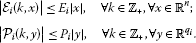(11)

The main problem here is to find a positive number γ such that the zero solution of an arbitrary perturbed system of the form ( 9 ) remains exponentially stable whenever the size of perturbations is less than γ.

Remark 2.4 In particular, if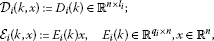and

${\mathcal{P}}_{i}\left(k,y\right):={P}_{i}\left(k\right)y,\phantom{\rule{1em}{0ex}}{P}_{i}\left(k\right)\in {\mathbb{R}}^{{l}_{i}×{q}_{i}},y\in {\mathbb{R}}^{{q}_{i}},$

then the perturbation ${\sum }_{i=1}^{N}{\mathcal{D}}_{i}\left(k,x\left(k\right)\right){\mathcal{P}}_{i}\left(k,{\mathcal{E}}_{i}\left(k,x\left(k\right)\right)\right)$ becomes ${\sum }_{i=1}^{N}{D}_{i}\left(k\right){P}_{i}\left(k\right){E}_{i}\left(k\right)x\left(k\right)$. The problem of robust stability of linear infinite dimensional time-varying system

$x\left(k+1\right)=A\left(k\right)x\left(k\right),\phantom{\rule{1em}{0ex}}k\in {\mathbb{Z}}_{+},$
(12)

under the time-varying multi-perturbations

$A\left(k\right)↪A\left(k\right)+\sum _{i=1}^{N}{D}_{i}\left(k\right){P}_{i}\left(k\right){E}_{i}\left(k\right),$
(13)

has been analyzed in  and an abstract stability bound is given in terms of input-output operators.

We are now in the position to prove the main result of this paper.

Theorem 2.5 Assume that all hypotheses of Proposition 2.2 hold and$A\in {\mathbb{R}}_{+}^{n×n}$satisfies (5). If (${H}_{1}$)-(${H}_{2}$) hold and

$\sum _{i=1}^{N}\parallel {P}_{i}\parallel <\frac{1}{{max}_{i,j\in \underline{N}}\parallel {E}_{i}{\left({I}_{n}-A\right)}^{-1}{D}_{j}\parallel },$
(14)

then the zero solution of (9) remains exponentially stable.

Proof Since (5) and (10)-(11), it follows that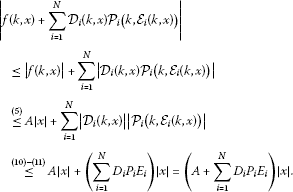We show that $\rho \left(A+{\sum }_{i=1}^{N}{D}_{i}{P}_{i}{E}_{i}\right)<1$ and then the zero solution of (9) is exponentially stable by Proposition 2.2.

Since A and ${D}_{i}$, ${E}_{i}$, ${P}_{i}$ ($i\in \underline{N}$) are nonnegative, so is $A+{\sum }_{i=1}^{N}{D}_{i}{P}_{i}{E}_{i}$. Assume on the contrary that ${\rho }_{0}:=\rho \left(A+{\sum }_{i=1}^{N}{D}_{i}{P}_{i}{E}_{i}\right)\ge 1$. By the Perron-Frobenius Theorem (Theorem 1.1(i)), there exists $x\in {\mathbb{R}}_{+}^{n}$, $x\ne 0$ such that

$\left(A+\sum _{i=1}^{N}{D}_{i}{P}_{i}{E}_{i}\right)x={\rho }_{0}x.$

Let $Q\left(t\right):=t{I}_{n}-A$, $t\in \mathbb{R}$. Since $\rho \left(A\right)<1$, $Q\left({\rho }_{0}\right)$ is invertible. It follows that

$Q{\left({\rho }_{0}\right)}^{-1}\sum _{i=1}^{N}{D}_{i}{P}_{i}{E}_{i}x=x.$
(15)

Let ${i}_{0}$ be an index such that $\parallel {E}_{{i}_{0}}x\parallel ={max}_{i\in \underline{N}}\parallel {E}_{i}x\parallel$. Then (15) implies that $\parallel {E}_{{i}_{0}}x\parallel >0$. Multiply both sides of (15) from the left by ${E}_{{i}_{0}}$, to get

$\sum _{i=1}^{N}{E}_{{i}_{0}}Q{\left({\rho }_{0}\right)}^{-1}{D}_{i}{P}_{i}{E}_{i}x={E}_{{i}_{0}}x.$

Taking norms, we get

$\sum _{i=1}^{N}\parallel {E}_{{i}_{0}}Q{\left({\rho }_{0}\right)}^{-1}{D}_{i}\parallel \parallel {P}_{i}\parallel \parallel {E}_{i}x\parallel \ge \parallel {E}_{{i}_{0}}x\parallel .$

This implies

$\underset{i,j\in \underline{N}}{max}\parallel {E}_{i}Q{\left({\rho }_{0}\right)}^{-1}{D}_{j}\parallel \left(\sum _{i=1}^{N}\parallel {P}_{i}\parallel \right)\parallel {E}_{{i}_{0}}x\parallel \ge \parallel {E}_{{i}_{0}}x\parallel ,$

or equivalently,

$\underset{i,j\in \underline{N}}{max}\parallel {E}_{i}Q{\left({\rho }_{0}\right)}^{-1}{D}_{j}\parallel \sum _{i=1}^{N}\parallel {P}_{i}\parallel \ge 1.$
(16)

On the other hand, the resolvent identity gives

$Q{\left(1\right)}^{-1}-Q{\left({\rho }_{0}\right)}^{-1}=\left({\rho }_{0}-1\right)Q{\left(1\right)}^{-1}Q{\left({\rho }_{0}\right)}^{-1}.$
(17)

Since $A\in {\mathbb{R}}_{+}^{n×n}$ and $\rho \left(A\right)<1\le {\rho }_{0}$, Theorem 1.1(iii) yields $Q{\left(1\right)}^{-1}\ge 0$ and $Q{\left({\rho }_{0}\right)}^{-1}\ge 0$. Then (17) implies $Q{\left(1\right)}^{-1}\ge Q{\left({\rho }_{0}\right)}^{-1}\ge 0$. Hence, ${E}_{i}Q{\left(1\right)}^{-1}{D}_{j}\ge {E}_{i}Q{\left({\rho }_{0}\right)}^{-1}{D}_{j}\ge 0$, for any $i,j\in \underline{N}$. By (1), $\parallel {E}_{i}Q{\left(1\right)}^{-1}{D}_{j}\parallel \ge \parallel {E}_{i}Q{\left({\rho }_{0}\right)}^{-1}{D}_{j}\parallel$, for any $i,j\in \underline{N}$. It follows from (16) that

$\sum _{i=1}^{N}\parallel {P}_{i}\parallel \ge \frac{1}{{max}_{i,j\in \underline{N}}\parallel {E}_{i}Q{\left(1\right)}^{-1}{D}_{j}\parallel }.$

However, this conflicts with (14). This completes the proof. □

In particular, suppose (12) satisfies

$|A\left(k\right)|\le A,\phantom{\rule{1em}{0ex}}\mathrm{\forall }k\in {\mathbb{Z}}_{+},$
(18)

for some $A\in {\mathbb{R}}_{+}^{n×n}$. Consider a perturbed system of the form

$x\left(k+1\right)=A\left(k\right)x\left(k\right)+\sum _{i=1}^{N}{\mathcal{D}}_{i}\left(k,x\left(k\right)\right){\mathcal{P}}_{i}\left(k,{\mathcal{E}}_{i}\left(k,x\left(k\right)\right)\right),\phantom{\rule{1em}{0ex}}k\in {\mathbb{Z}}_{+},$
(19)

where ${\mathcal{D}}_{i}$, ${\mathcal{P}}_{i}$ and ${\mathcal{E}}_{i}$ ($i\in \underline{N}$) are as above.

The following is immediate from Theorem 2.5.

Corollary 2.6 Suppose (18) and (${H}_{1}$)-(${H}_{2}$) hold and$\rho \left(A\right)<1$. If (14) holds then the zero solution of (19) is exponentially stable.

Corollary 2.7 Let$A\in {\mathbb{R}}_{+}^{n×n}$and$\rho \left(A\right)<1$. Suppose${D}_{i}\left(\cdot \right):{\mathbb{Z}}_{+}\to {\mathbb{R}}^{n×{l}_{i}}$, ${E}_{i}\left(\cdot \right):{\mathbb{Z}}_{+}\to {\mathbb{R}}^{{q}_{i}×n}$ ($i\in \underline{N}$), are given and${P}_{i}\left(\cdot \right):{\mathbb{Z}}_{+}\to {\mathbb{R}}^{{l}_{i}×{q}_{i}}$ ($i\in \underline{N}$) are unknown. If there exist${D}_{i}\in {\mathbb{R}}^{n×{l}_{i}}$, ${E}_{i}\in {\mathbb{R}}^{{q}_{i}×n}$and${P}_{i}\in {\mathbb{R}}^{{l}_{i}×{q}_{i}}$ ($i\in \underline{N}$) such that

$|{D}_{i}\left(k\right)|\le {D}_{i};\phantom{\rule{2em}{0ex}}|{E}_{i}\left(k\right)|\le {E}_{i};\phantom{\rule{2em}{0ex}}|{P}_{i}\left(k\right)|\le {P}_{i},\phantom{\rule{1em}{0ex}}\mathrm{\forall }k\in {\mathbb{Z}}_{+},$

and (14) holds then the zero solution of the perturbed system

$x\left(k+1\right)=\left(A+\sum _{i=1}^{N}{D}_{i}\left(k\right){P}_{i}\left(k\right){E}_{i}\left(k\right)\right)x\left(k\right),\phantom{\rule{1em}{0ex}}\mathrm{\forall }k\in {\mathbb{Z}}_{+},$
(20)

is exponentially stable.

Remark 2.8 If $A\in {\mathbb{R}}_{+}^{n×n}$, then the system

$x\left(k+1\right)=Ax\left(k\right),\phantom{\rule{1em}{0ex}}k\in {\mathbb{Z}}_{+},$
(21)

is positive. That is, for any initial state ${x}_{0}\in {\mathbb{R}}_{+}^{n}$, the corresponding trajectory of the system $x\left(k,{x}_{0}\right)$, $k\in {\mathbb{Z}}_{+}$, remains in ${\mathbb{R}}_{+}^{n}$ for all $k\in {\mathbb{Z}}_{+}$. Positive dynamical systems play an important role in the modeling of dynamical phenomena whose variables are restricted to be nonnegative. They are often encountered in applications, for example, networks of reservoirs, industrial processes involving chemical reactors, heat exchangers, distillation columns, storage systems, hierarchical systems, compartmental systems used for modeling transport and accumulation phenomena of substances, see, e.g., [6, 10, 13].

In particular, the problem of robust stability of the positive linear discrete-time system (21) under the time-invariant structured perturbations

$A↪A+DPE,$

has been studied in [12, 19]. More precisely, it has been shown in [12, 19] that if (21) is exponentially stable and positive and D, E are given nonnegative matrices then a perturbed system of the form

$x\left(k+1\right)=\left(A+DPE\right)x\left(k\right),\phantom{\rule{1em}{0ex}}k\in {\mathbb{Z}}_{+},$

remains exponentially stable whenever

$\parallel P\parallel <\frac{1}{\parallel E{\left({I}_{n}-A\right)}^{-1}D\parallel }.$

Furthermore, the problem of robust stability of the positive system (21) under the time-invariant multi-perturbations

$A↪A+\sum _{i=1}^{N}{D}_{i}{P}_{i}{E}_{i},$

has been analyzed in  by techniques of μ-analysis.

Although there are many works devoted to the study of robust stability of discrete-time systems, to the best of our knowledge, the problem of robust stability of the positive system (21) under the time-varying multi-perturbations

$A↪A+\sum _{i=1}^{N}{D}_{i}\left(k\right){P}_{i}\left(k\right){E}_{i}\left(k\right),$

has not been studied yet, and a result like Corollary 2.7 cannot be found in the literature.

We illustrate the obtained results by a couple of examples.

Example 2.9 Consider the nonlinear time-varying equation

$x\left(k+1\right)=\frac{1}{4}{e}^{-k}x\left(k\right)+sin\left(\frac{k}{{k}^{2}+1}x\left(k\right)\right),\phantom{\rule{1em}{0ex}}k\in {\mathbb{Z}}_{+}.$
(22)

Clearly, (22) is of the form (2) with $f\left(k,x\right):=\frac{1}{4}{e}^{-k}x+sin\left(\frac{k}{{k}^{2}+1}x\right)$. Since

$|f\left(k,x\right)|=|\frac{1}{4}{e}^{-k}x+sin\left(\frac{k}{{k}^{2}+1}x\right)|\le \frac{1}{4}|x|+|\frac{k}{{k}^{2}+1}x|\le \frac{3}{4}|x|,\phantom{\rule{1em}{0ex}}\mathrm{\forall }k\in {\mathbb{Z}}_{+},\mathrm{\forall }x\in \mathbb{R},$

the zero solution of (22) is exponentially stable, by Proposition 2.2.

Consider a perturbed equation given by

$x\left(k+1\right)=\left(\frac{1}{4}{e}^{-k}+a{e}^{-{k}^{2}-1}\right)x\left(k\right)+sin\left(\frac{k}{{k}^{2}+1}x\left(k\right)\right)+arctan\left(bx\left(k\right)\right),\phantom{\rule{1em}{0ex}}k\in {\mathbb{Z}}_{+},$
(23)

where $a,b\in \mathbb{R}$ are parameters.

Note that $|a{e}^{-{k}^{2}-1}x|\le {e}^{-1}|a||x|$ and $|arctan\left(bx\right)|\le |b||x|$, for all $k\in {\mathbb{Z}}_{+}$, $x\in \mathbb{R}$. By Theorem 2.5, the zero solution of (23) is exponentially stable if ${e}^{-1}|a|+|b|<\frac{1}{4}$.

Example 2.10 Consider a linear discrete-time equation in ${\mathbb{R}}^{2}$ defined by

$x\left(k+1\right)=Ax\left(k\right),\phantom{\rule{1em}{0ex}}k\in {\mathbb{Z}}_{+},$
(24)

where

$A:=\left(\begin{array}{cc}\frac{1}{2}& \frac{1}{2}\\ \frac{1}{4}& \frac{1}{2}\end{array}\right).$

Clearly, (24) is positive and exponentially stable. Consider a perturbed system given by

$x\left(k+1\right)=\left(A+{D}_{1}\left(k\right){P}_{1}\left(k\right){E}_{1}\left(k\right)+{D}_{2}\left(k\right){P}_{2}\left(k\right){E}_{2}\left(k\right)\right)x\left(k\right),\phantom{\rule{1em}{0ex}}k\in {\mathbb{Z}}_{+},$
(25)

where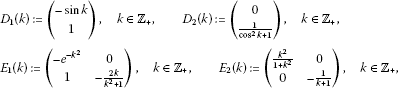and ${P}_{1}\left(k\right):=\left(a\left(k\right),b\left(k\right)\right)\in {\mathbb{R}}^{1×2}$; ${P}_{2}\left(k\right):=\left(c\left(k\right),d\left(k\right)\right)\in {\mathbb{R}}^{1×2}$, $k\in {\mathbb{Z}}_{+}$ are unknown perturbations.

Note that for any $k\in {\mathbb{Z}}_{+}$, we have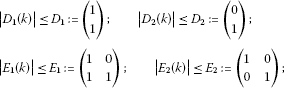and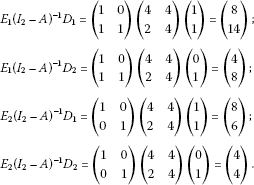Let ${\mathbb{R}}^{2}$ be endowed with 2-norm. By Corollary 2.7, (25) is exponentially stable provided

$\sqrt{{\left(\underset{k\in {\mathbb{Z}}_{+}}{sup}|a\left(k\right)|\right)}^{2}+{\left(\underset{k\in {\mathbb{Z}}_{+}}{sup}|b\left(k\right)|\right)}^{2}}+\sqrt{{\left(\underset{k\in {\mathbb{Z}}_{+}}{sup}|c\left(k\right)|\right)}^{2}+{\left(\underset{k\in {\mathbb{Z}}_{+}}{sup}|d\left(k\right)|\right)}^{2}}<\frac{1}{2\sqrt{65}}.$

## 3 Aizerman-type problem

As an application, we now deal with an Aizerman-type problem for discrete-time systems.

Aizerman-type conjecture for discrete-time systems (ATC-DTS) Let$A\in {\mathbb{R}}^{n×n}$, $D\in {\mathbb{R}}^{n×l}$, $E\in {\mathbb{R}}^{q×n}$be given. For any$\gamma >0$the linear systems

$x\left(k+1\right)=\left(A+DPE\right)x\left(k\right),\phantom{\rule{1em}{0ex}}P\in {\mathbb{R}}^{l×q},\parallel P\parallel <\gamma ,$
(26)

are asymptotically stable if and only if the origin is globally asymptotically stable for all nonlinear systems

$x\left(k+1\right)=Ax\left(k\right)+D\mathcal{N}\left(k,Ex\left(k\right)\right),$
(27)

where$\mathcal{N}:{\mathbb{Z}}_{+}×{\mathbb{R}}^{q}\to {\mathbb{R}}^{l}$, $\mathcal{N}\left(k,0\right)=0$, $\mathrm{\forall }k\in {\mathbb{Z}}_{+}$, satisfies

$|\mathcal{N}\left(k,y\right)|\le P|y|,\phantom{\rule{1em}{0ex}}\mathrm{\forall }k\in {\mathbb{Z}}_{+},\mathrm{\forall }y\in {\mathbb{R}}^{q}\mathit{\text{and}}P\in {\mathbb{R}}^{l×q},\parallel P\parallel <\gamma .$
(28)

In particular, when $\mathcal{N}:\mathbb{R}\to \mathbb{R}$, $y↦\mathcal{N}\left(y\right)$, is a scalar function and $D,{E}^{T}\in {\mathbb{R}}^{n}$, the above conjecture is exactly a discrete-time version of the original Aizerman conjecture which was formulated first for ordinary differential systems, see . It is well known that in general, the Aizerman classical conjecture does not hold, see, e.g., . So a natural question arising here is that under what conditions of A, D, E and $\mathcal{N}$ does the ATC-DTS hold?

Theorem 3.1 If$A\in {\mathbb{R}}_{+}^{n×n}$and$D\in {\mathbb{R}}_{+}^{n×l}$, $E\in {\mathbb{R}}_{+}^{q×n}$then the ATC-DTS holds.

In other words, the ATC-DTS holds for positive systems.

Proof Suppose (26) is asymptotically stable for any $P\in {\mathbb{R}}^{l×q}$, $\parallel P\parallel <\gamma$, for some $\gamma >0$. In particular, the unperturbed system (21) is asymptotically stable. It follows from Corollary 2.7 that (26) is asymptotically stable for any $P\in {\mathbb{R}}^{l×q}$, $\parallel P\parallel <\frac{1}{\parallel E{\left({I}_{n}-A\right)}^{-1}D\parallel }$ (see also Remark 2.8). Furthermore, there exists ${P}_{0}\in {\mathbb{R}}_{+}^{l×q}$, $\parallel {P}_{0}\parallel =\frac{1}{\parallel E{\left({I}_{n}-A\right)}^{-1}D\parallel }$ such that (26) is not asymptotically stable for $P:={P}_{0}$, see, e.g., [12, 19]. It remains to show that the zero solution of (27) is globally asymptotically stable for any nonlinearity $\mathcal{N}$ satisfying (28) with $\gamma :=\frac{1}{\parallel E{\left({I}_{n}-A\right)}^{-1}D\parallel }$. Let $\mathcal{N}$ satisfy (28) with $\gamma :=\frac{1}{\parallel E{\left({I}_{n}-A\right)}^{-1}D\parallel }$. Since $P\in {\mathbb{R}}^{l×q}$, $\parallel P\parallel <\frac{1}{\parallel E{\left({I}_{n}-A\right)}^{-1}D\parallel }$, the zero solution of (27) is globally asymptotically stable, by Corollary 2.6.

Conversely, assume that the zero solution of (27) is globally asymptotically stable for any nonlinearity $\mathcal{N}$ satisfying (28) for some $\gamma >0$. Then the unperturbed system (21) is asymptotically stable. As mentioned above, (26) is asymptotically stable for any $P\in {\mathbb{R}}^{l×q}$, $\parallel P\parallel <\frac{1}{\parallel E{\left({I}_{n}-A\right)}^{-1}D\parallel }$. So we assume that $\gamma \ge \frac{1}{\parallel E{\left({I}_{n}-A\right)}^{-1}D\parallel }$. Note that (26) is not asymptotically stable for some ${P}_{0}\in {\mathbb{R}}_{+}^{l×q}$, $\parallel {P}_{0}\parallel =\frac{1}{\parallel E{\left({I}_{n}-A\right)}^{-1}D\parallel }$. This means that the zero solution of (27) is not globally asymptotically stable for $\mathcal{N}$ defined by $\mathcal{N}\left(k,y\right):={P}_{0}y$, $k\in {\mathbb{Z}}_{+}$, $y\in {\mathbb{R}}^{q}$. This completes the proof. □

Remark 3.2 In general, the question ‘Under what conditions of A, D, E and $\mathcal{N}$ does the ATC-DTS hold?’ is still open.

## References

1. Aizerman MA: On one problem concerning the stability in ‘large’ of dynamic systems. Usp. Mat. Nauk 1949, 4: 186–188.

2. Buslowicz M, Kaczorek T: Robust stability of positive discrete-time interval systems with time-delays. Bull. Pol. Acad. Sci., Tech. Sci. 2004, 52: 99–102.

3. Shyh-Feng C: Asymptotic stability of discrete-time systems with time-varying delay subject to saturation nonlinearities. Chaos Solitons Fractals 2009, 42: 1251–1257. 10.1016/j.chaos.2009.03.026

4. Dieudonné J: Foundations of Modern Analysis. Academic Press, San Diego; 1988.

5. Elaydi S: An Introduction to Difference Equations. 3rd edition. Springer, Berlin; 2005.

6. Farina L, Rinaldi S: Positive Linear Systems: Theory and Applications. Wiley, New York; 2000.

7. Fitts RE: Two counterexamples to Aizerman’s conjecture. IEEE Trans. Autom. Control 1966, 11: 553–556. 10.1109/TAC.1966.1098369

8. Fiedler M, Pták V: On matrices with non-positive off-diagonal elements and positive principal minors. Czechoslov. Math. J. 1962, 12: 382–400.

9. Haddad WM, Chellaboina V, August E: Stability and dissipativity theory for discrete-time nonnegative and compartmental dynamical systems. Int. J. Control 2003, 76: 1845–1861. 10.1080/00207170310001635400

10. Haddad WM, Chellaboina V, Hui Q: Nonnegative and Compartmental Dynamical Systems. Princeton University Press, Princeton; 2010.

11. Hinrichsen D, Pritchard AJ: Mathematical Systems Theory I. Springer, Berlin; 2005.

12. Hinrichsen D, Son NK: Stability radii of positive discrete-time systems under affine parameter perturbations. Int. J. Robust Nonlinear Control 1998, 8: 1169–1188. 10.1002/(SICI)1099-1239(1998110)8:13<1169::AID-RNC381>3.0.CO;2-#

13. Kaczorek T: Positive 1D and 2D Systems. Springer, London; 2002.

14. Kolmanovskii VB, Castellanos-Velasco E, Torres-Munoz JA: A survey: stability and boundedness of Volterra difference equations. Nonlinear Anal. 2003, 53: 861–928. 10.1016/S0362-546X(03)00021-X

15. Mickens RE: Difference Equations: Theory and Applications. Chapman & Hall, London; 1990.

16. Ngoc PHA, Son NK: Stability radii of positive linear difference equations under affine parameter perturbations. Appl. Math. Comput. 2003, 134: 577–594. 10.1016/S0096-3003(01)00306-X

17. Ngoc PHA, Naito T, Shin JS, Murakami S: Stability and robust stability of positive linear Volterra difference equations. Int. J. Robust Nonlinear Control 2009, 19: 552–569. 10.1002/rnc.1335

18. Ramakrishnan, K, Ray, G: Robust stability criteria for a class of uncertain discrete-time systems with time-varying delay. Appl. Math. Model. (2012, in press). doi:10.1016/j.apm.2012.03.045

19. Shafai B, Chen J, Kothandaraman M: Explicit formulas for stability radii of nonnegative and Metzlerian matrices. IEEE Trans. Autom. Control 1997, 42: 265–270. 10.1109/9.554408

20. Singh V: Stability of discrete-time systems joined with a saturation operator on the state space: generalized form of Liu-Michel’s criterion. Automatica 2011, 47: 634–637. 10.1016/j.automatica.2011.01.041

21. Wirth, F: Robust stability of discrete-time systems under time-varying perturbations. PhD thesis, Bremen University, Bremen (1995)

## Author information

Authors

### Corresponding author

Correspondence to Pham Huu Anh Ngoc.

### Competing interests

The authors declare that they have no competing interests.

### Authors’ contributions

All authors contributed equally and significantly in writing this article. All authors read and approved the final manuscript.

## Rights and permissions

Reprints and Permissions

Ngoc, P.H.A., Hieu, L.T. On stability of discrete-time systems under nonlinear time-varying perturbations. Adv Differ Equ 2012, 120 (2012). https://doi.org/10.1186/1687-1847-2012-120